# Predicting Yields In A Tuna Cannery Using Machine Learning

Nov 10, 2020BY APARNA KORATTYSWAROOPAM, LEAD DATA SCIENTIST, THISFISH INC.

Among the biggest decision-drivers in the food industry is the yield – defined as the ratio of usable output to the raw material input. Researchers have been using statistics and food science to understand what impacts yields for over a century now.

With machine learning and increased data, we now have the ability to predict yields with significant precision.

At ThisFish, we have been running a research project in collaboration with York University’s researchers to understand the best predictor of yields in a tuna cannery. We see very interesting results that we will talk about in our upcoming newsletters.

In this article, we will explore the elementary ideas and challenges in the application of Machine Learning using the example of Yield prediction in a tuna cannery.

# Machine Learning – A Primer

Before we delve into a real-world application of machine learning, it would help to look at what It means to “train” a machine learning model. If we were to peek below the hood, the steps involved in “training” a machine learning model would boil down to these four steps –

1.     Look at historical data for predictors and predictions

2.     Make guesses for new predictions

3.     Calculate the difference between the model predictions and actual predictions as errors

4.     Re-adjust guesses based on errors – repeat until the errors are as low as possible

To understand this better, let’s look at a set of data points as an example –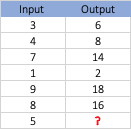Can you guess the last output in the table above?

Most of us probably saw a pattern here, which is –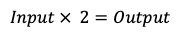This recognition of pattern comes to our brain from years of schooling (training).

When we train a machine learning model, we are training a computer to see a pattern the way we do.

In the example above, the value “2” is what is called a coefficient. This is the value that a machine learning model tries “learn”.

The first step to learning is a random guess. Say the model takes a random guess of 11 as the value of the coefficient. So, the model now predicts the following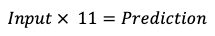It predicts the following output for all the known values of data and calculates the errors as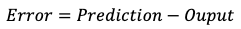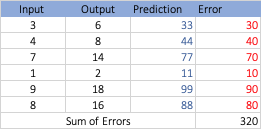The model now looks at the “Sum of Errors” and decides it probably guessed the coefficient way too high. So, in the next step, it reduces the coefficient to say, 5 and see the following output, i.e.,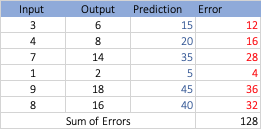It sees that the sum of errors has reduced, so it now knows that reducing the coefficient was the right thing to do. So, in the next step it would reduce the coefficient even further, eventually arriving at the correct answer which is “2”.

# Yield prediction

Real-world data is rarely as straightforward as the example above.

When it comes to predicting yields, there are a lot of factors that can affect the outcome. From the waters in which the fish was caught, to the time for which it was frozen has an impact on the final yield.

Among all the factors that can affect yields, there are controllable factors and uncontrollable factors. For example, the waters in which the fish were caught, or the size and species of the fish are uncontrollable factors as far as a tuna cannery is concerned. However, factors such as the cooking time or freezing time are controllable factors.

Taking into account all types of factors, we were able to build a Machine Learning model that predicts the yields with a very high accuracy. The quality of a model such as a yield prediction model is measured using a number called the R2 score, ranging from 0 – 1, on which we have been able to achieve a score of 0.88 using a machine learning technique.

# Challenges

However, predicting yields is only one part of the problem. The more interesting question is what can we do to improve the yields? For this we will have to find relationships between yields and controllable factors

For example: Could we find a relationship between cooking times and yields such that we can say with good confidence that increasing the cooking time by a factor of 0.005 can lead to a 1% increase in yield overall? Drawing from the example above, this would mean that we find a relationship like this -

However, the relationships we would find may not be as simple as the one above. More often than not we might see a relationship that involves multiple factors that can affect yield. For example, we might find a relationship like –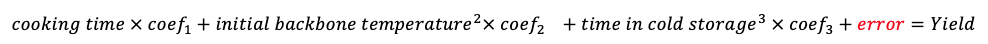Where the error component is used to catch all the hidden relationships we haven’t managed to uncover. It is because of such complexity among factors and yields that we have to go beyond the classic statistical methods and depend on more complex machine learning methods to uncover patterns.

# What’s next

We are seeing promising early results with our exploration of controllable factors that impact yield. Our next step is to develop a machine learning model that can help tuna canneries optimize their cooking process based on multiple variables. We hope to share results with you in the upcoming newsletters.